Скачать презентацию The Mass of a One Cent Coin T

3866f83b26175a89c04dec103d656eb8.ppt

• Количество слайдов: 29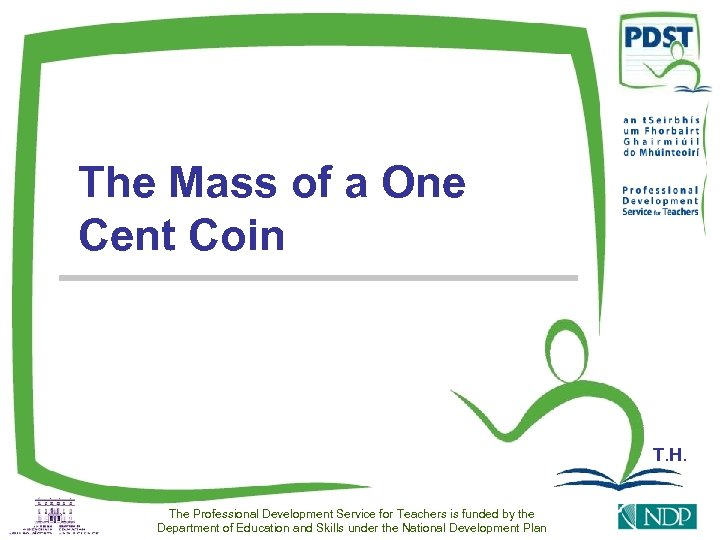The Mass of a One Cent Coin T. H. The Professional Development Service for Teachers is funded by the Department of Education and Skills under the National Development Plan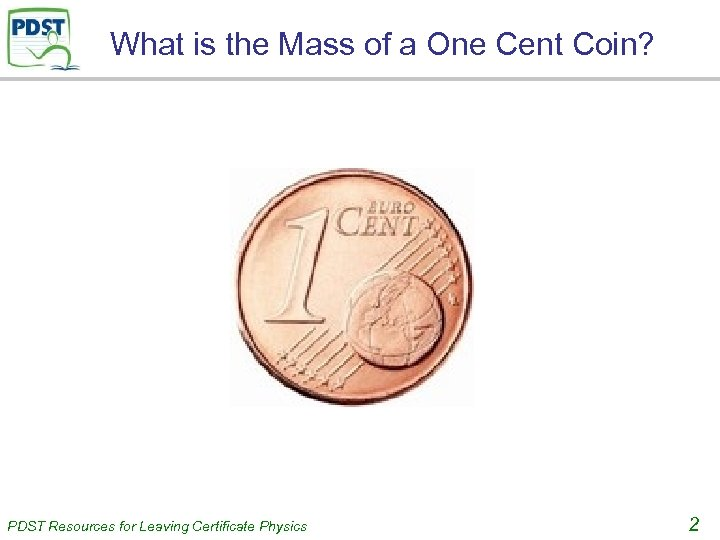What is the Mass of a One Cent Coin? PDST Resources for Leaving Certificate Physics 2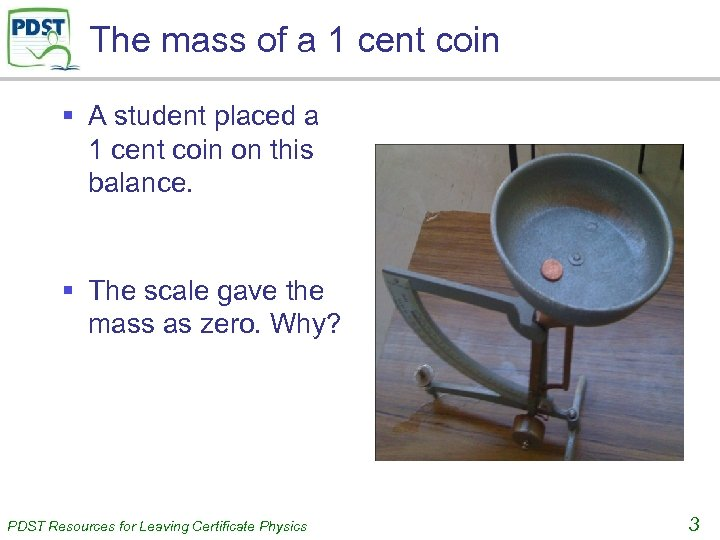The mass of a 1 cent coin § A student placed a 1 cent coin on this balance. § The scale gave the mass as zero. Why? PDST Resources for Leaving Certificate Physics 3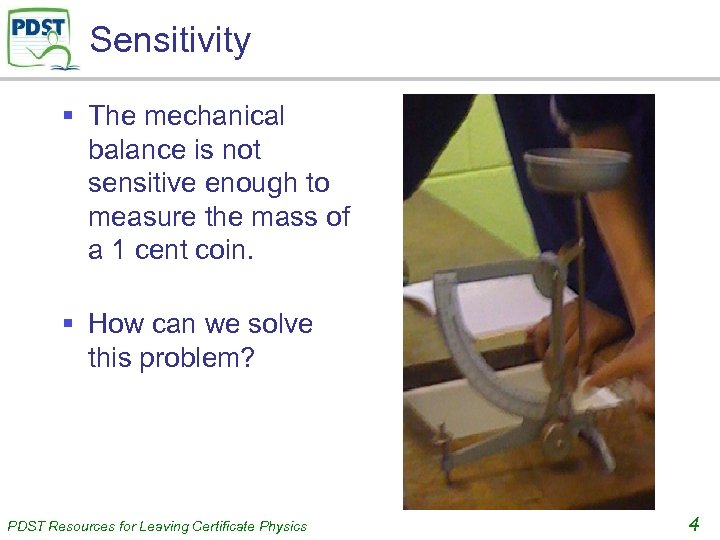Sensitivity § The mechanical balance is not sensitive enough to measure the mass of a 1 cent coin. § How can we solve this problem? PDST Resources for Leaving Certificate Physics 4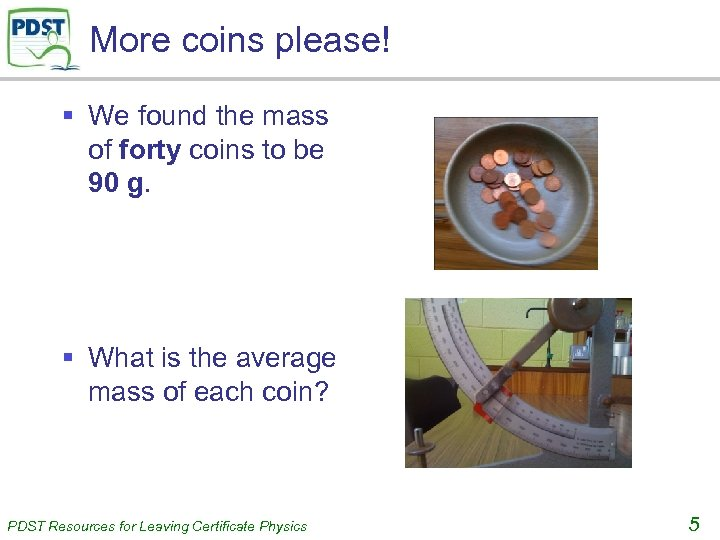More coins please! § We found the mass of forty coins to be 90 g. § What is the average mass of each coin? PDST Resources for Leaving Certificate Physics 5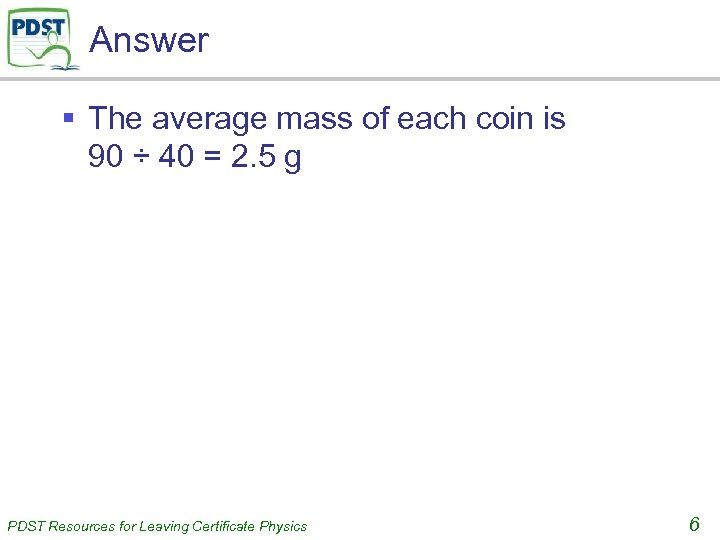Answer § The average mass of each coin is 90 ÷ 40 = 2. 5 g PDST Resources for Leaving Certificate Physics 6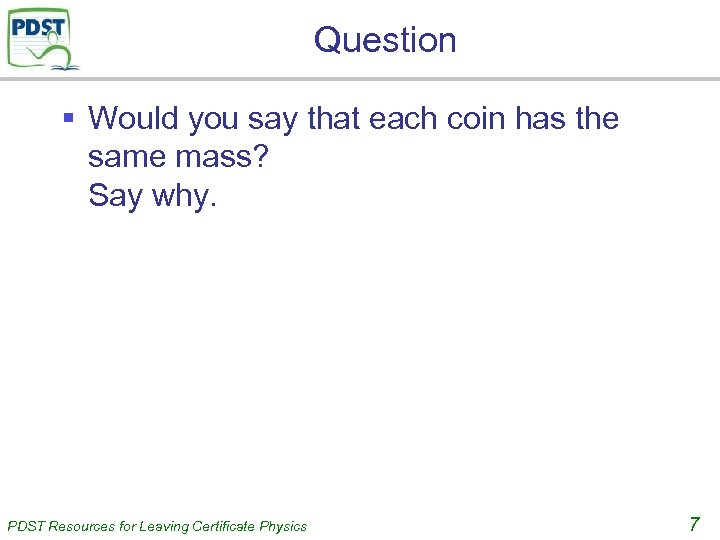Question § Would you say that each coin has the same mass? Say why. PDST Resources for Leaving Certificate Physics 7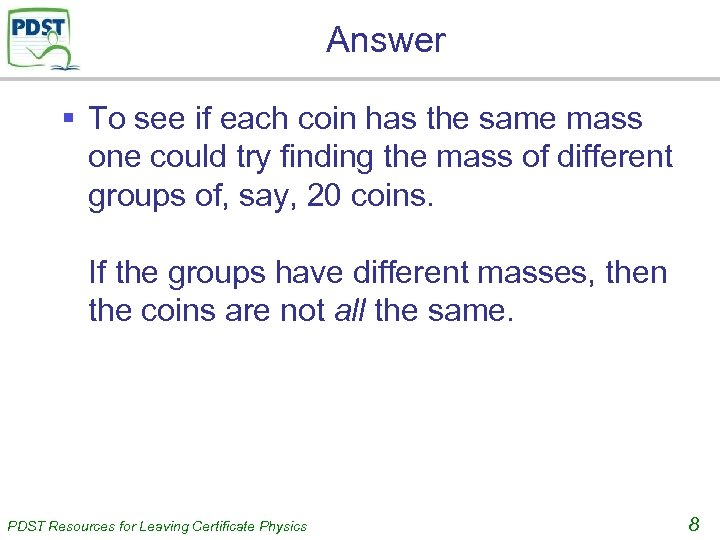Answer § To see if each coin has the same mass one could try finding the mass of different groups of, say, 20 coins. If the groups have different masses, then the coins are not all the same. PDST Resources for Leaving Certificate Physics 8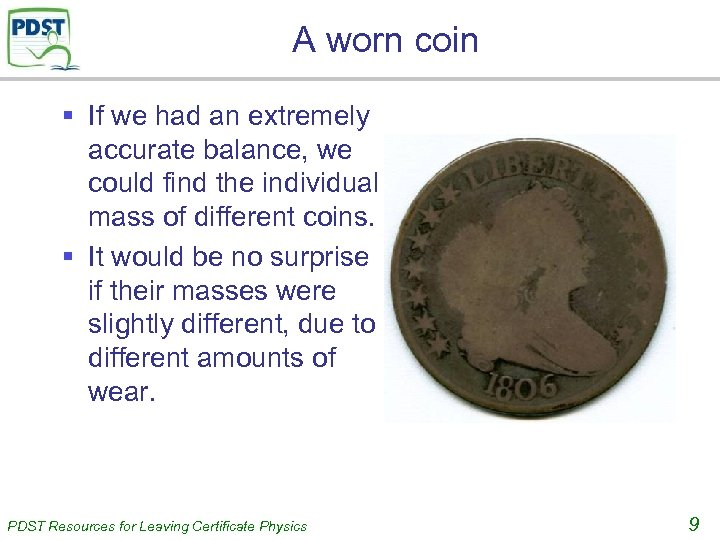A worn coin § If we had an extremely accurate balance, we could find the individual mass of different coins. § It would be no surprise if their masses were slightly different, due to different amounts of wear. PDST Resources for Leaving Certificate Physics 9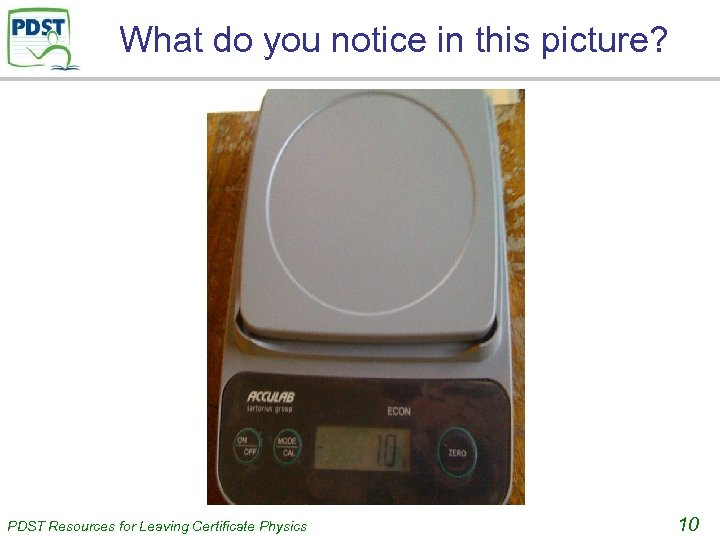What do you notice in this picture? PDST Resources for Leaving Certificate Physics 10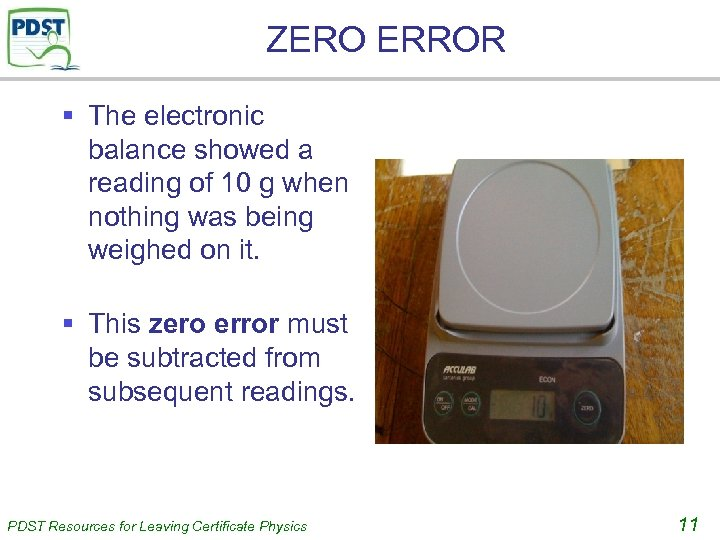ZERO ERROR § The electronic balance showed a reading of 10 g when nothing was being weighed on it. § This zero error must be subtracted from subsequent readings. PDST Resources for Leaving Certificate Physics 11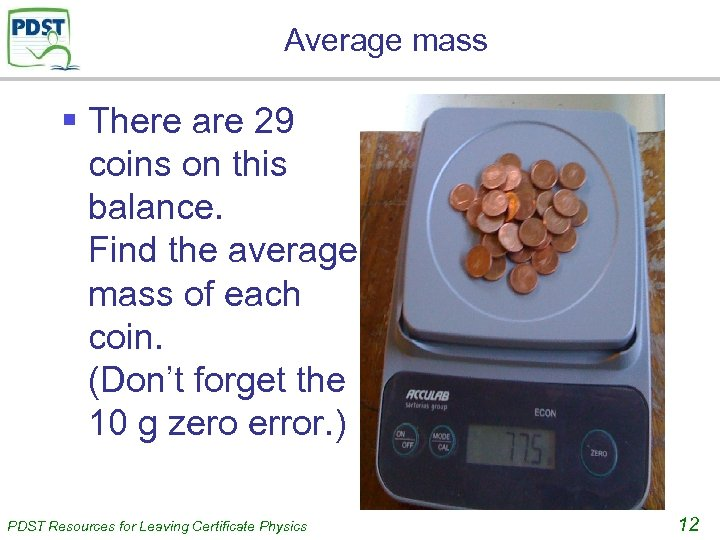Average mass § There are 29 coins on this balance. Find the average mass of each coin. (Don’t forget the 10 g zero error. ) PDST Resources for Leaving Certificate Physics 12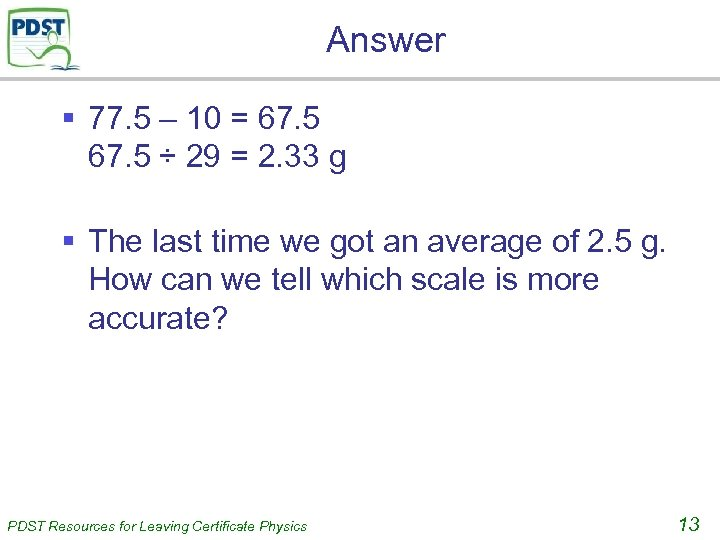Answer § 77. 5 – 10 = 67. 5 ÷ 29 = 2. 33 g § The last time we got an average of 2. 5 g. How can we tell which scale is more accurate? PDST Resources for Leaving Certificate Physics 13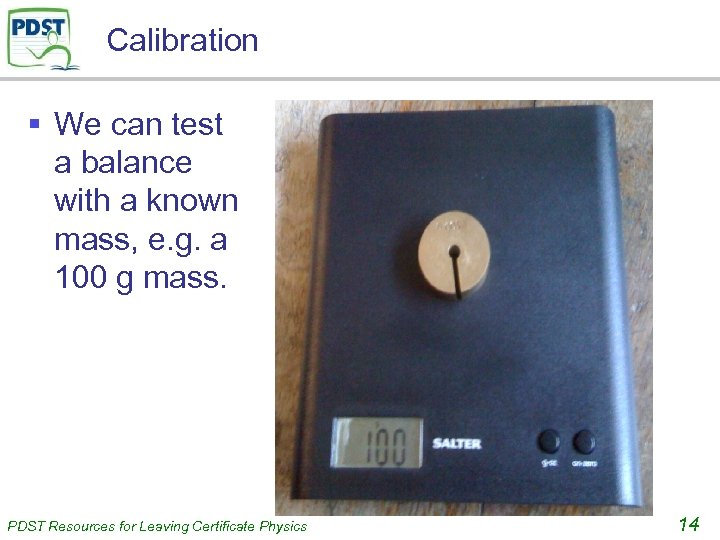Calibration § We can test a balance with a known mass, e. g. a 100 g mass. PDST Resources for Leaving Certificate Physics 14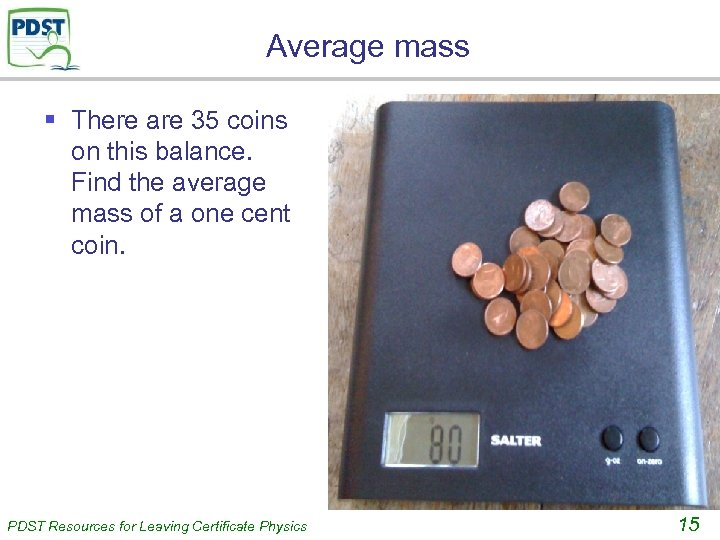Average mass § There are 35 coins on this balance. Find the average mass of a one cent coin. PDST Resources for Leaving Certificate Physics 15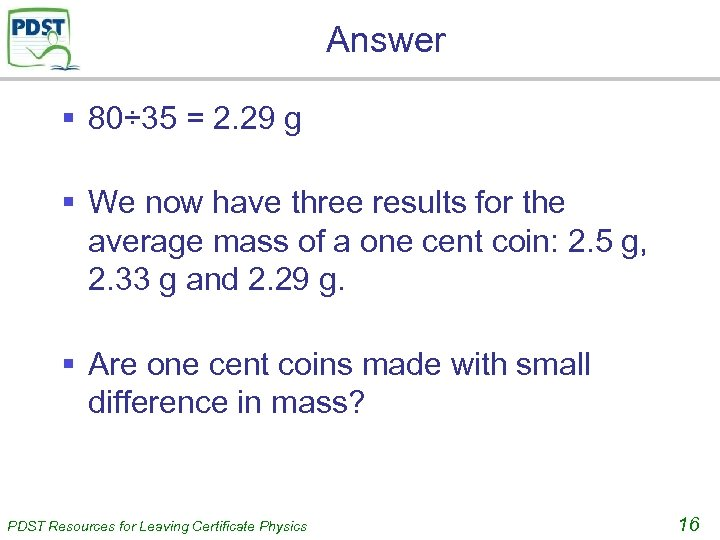Answer § 80÷ 35 = 2. 29 g § We now have three results for the average mass of a one cent coin: 2. 5 g, 2. 33 g and 2. 29 g. § Are one cent coins made with small difference in mass? PDST Resources for Leaving Certificate Physics 16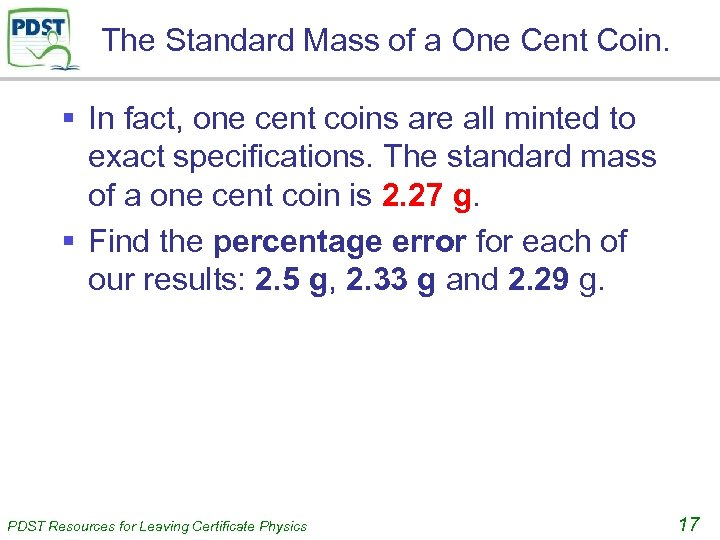The Standard Mass of a One Cent Coin. § In fact, one cent coins are all minted to exact specifications. The standard mass of a one cent coin is 2. 27 g. § Find the percentage error for each of our results: 2. 5 g, 2. 33 g and 2. 29 g. PDST Resources for Leaving Certificate Physics 17Percentage Errors PDST Resources for Leaving Certificate Physics 18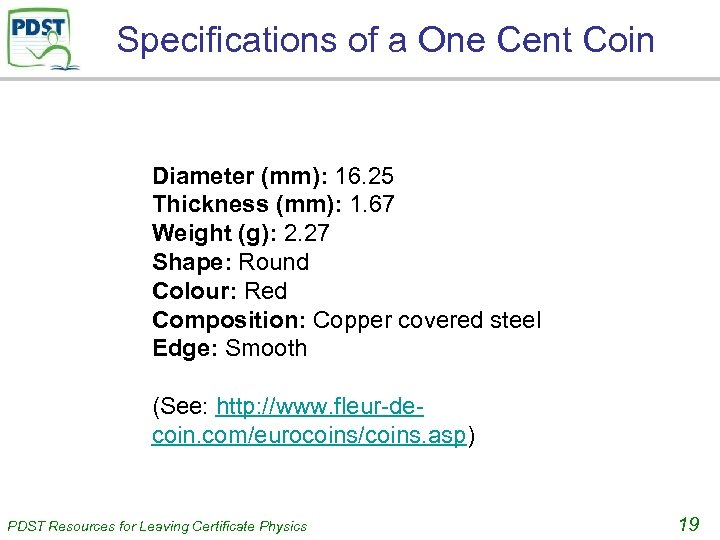Specifications of a One Cent Coin Diameter (mm): 16. 25 Thickness (mm): 1. 67 Weight (g): 2. 27 Shape: Round Colour: Red Composition: Copper covered steel Edge: Smooth (See: http: //www. fleur-decoin. com/eurocoins/coins. asp) PDST Resources for Leaving Certificate Physics 19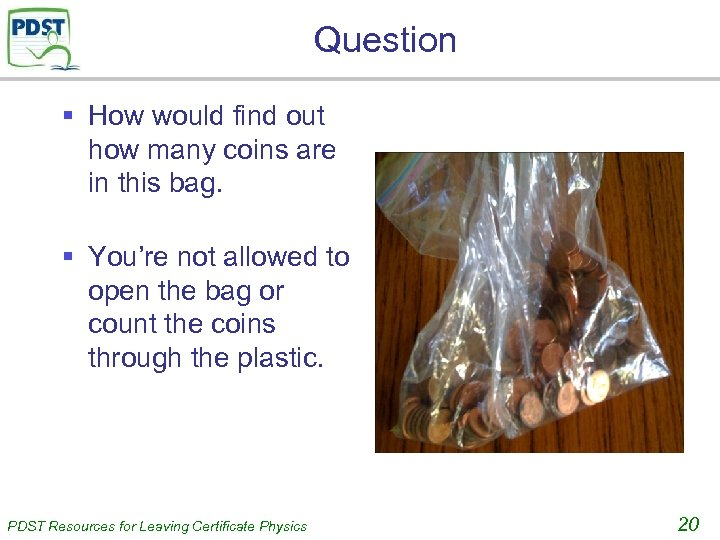Question § How would find out how many coins are in this bag. § You’re not allowed to open the bag or count the coins through the plastic. PDST Resources for Leaving Certificate Physics 20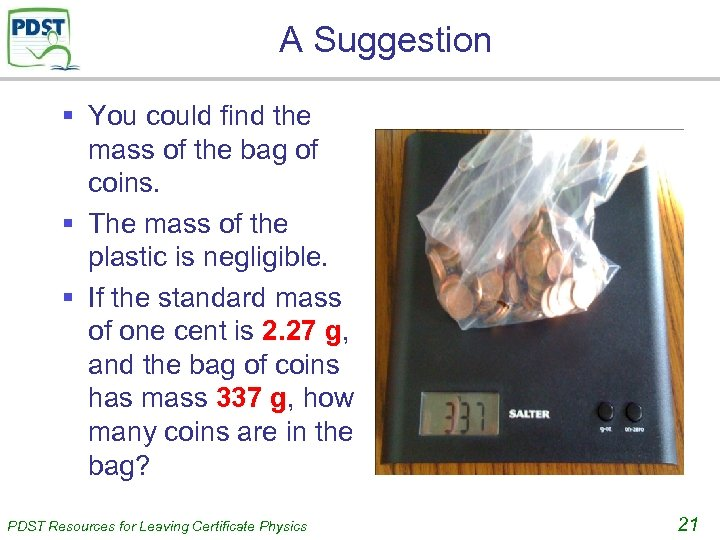A Suggestion § You could find the mass of the bag of coins. § The mass of the plastic is negligible. § If the standard mass of one cent is 2. 27 g, and the bag of coins has mass 337 g, how many coins are in the bag? PDST Resources for Leaving Certificate Physics 21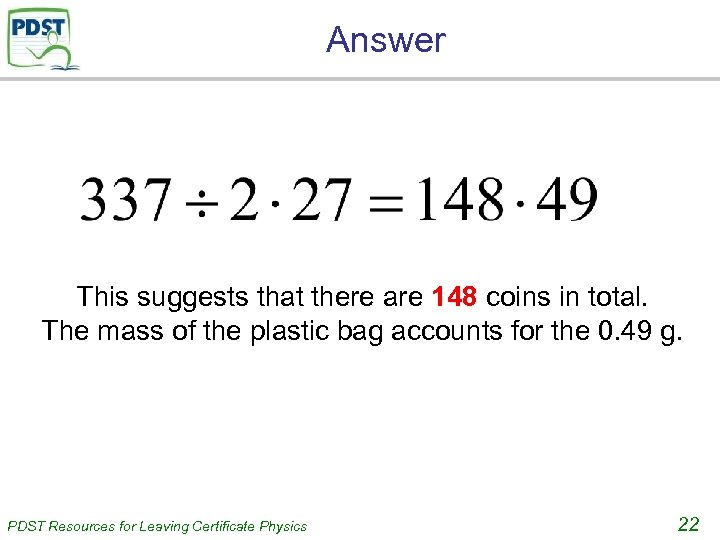Answer This suggests that there are 148 coins in total. The mass of the plastic bag accounts for the 0. 49 g. PDST Resources for Leaving Certificate Physics 22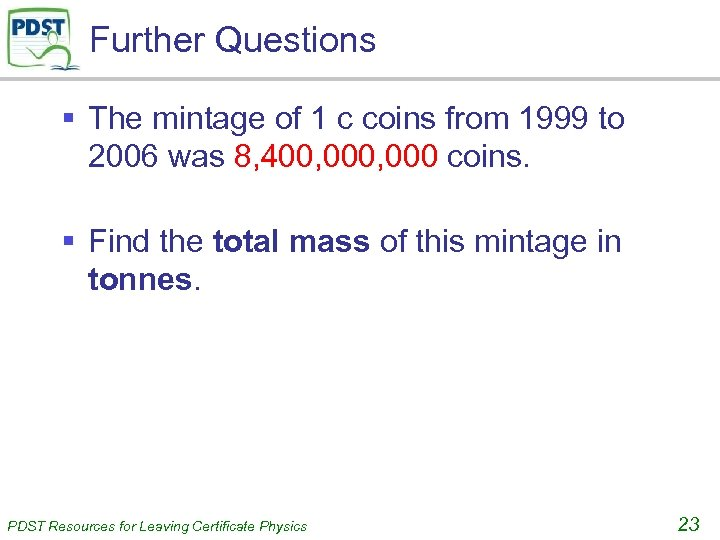Further Questions § The mintage of 1 c coins from 1999 to 2006 was 8, 400, 000 coins. § Find the total mass of this mintage in tonnes. PDST Resources for Leaving Certificate Physics 23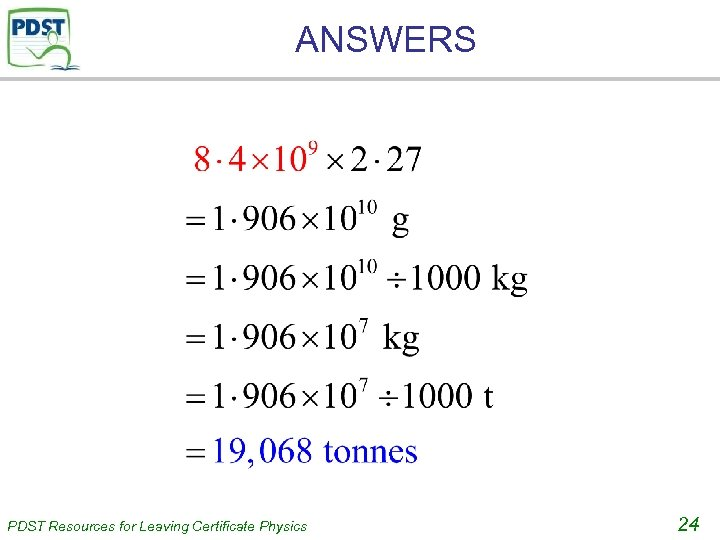ANSWERS PDST Resources for Leaving Certificate Physics 24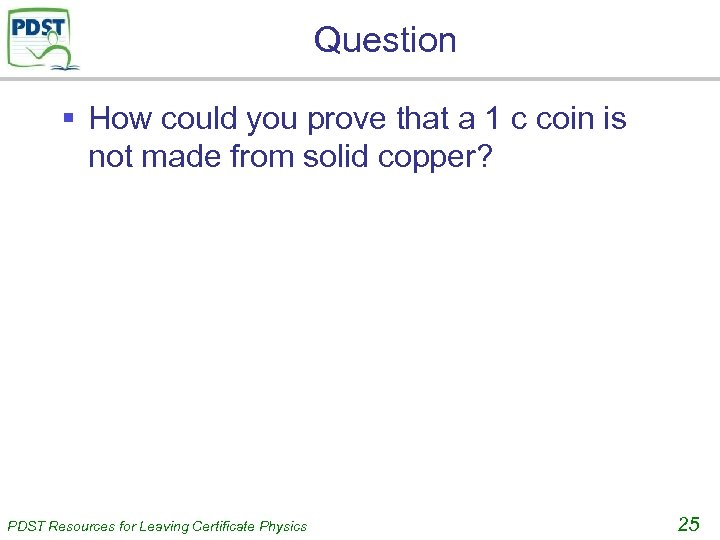Question § How could you prove that a 1 c coin is not made from solid copper? PDST Resources for Leaving Certificate Physics 25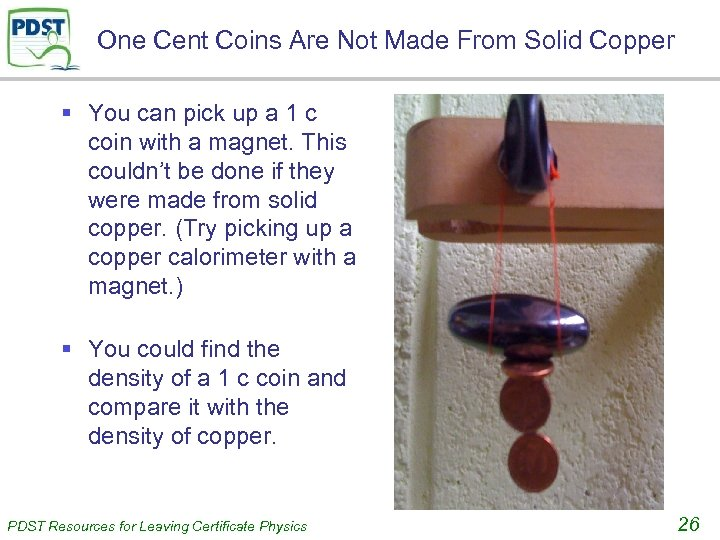One Cent Coins Are Not Made From Solid Copper § You can pick up a 1 c coin with a magnet. This couldn’t be done if they were made from solid copper. (Try picking up a copper calorimeter with a magnet. ) § You could find the density of a 1 c coin and compare it with the density of copper. PDST Resources for Leaving Certificate Physics 26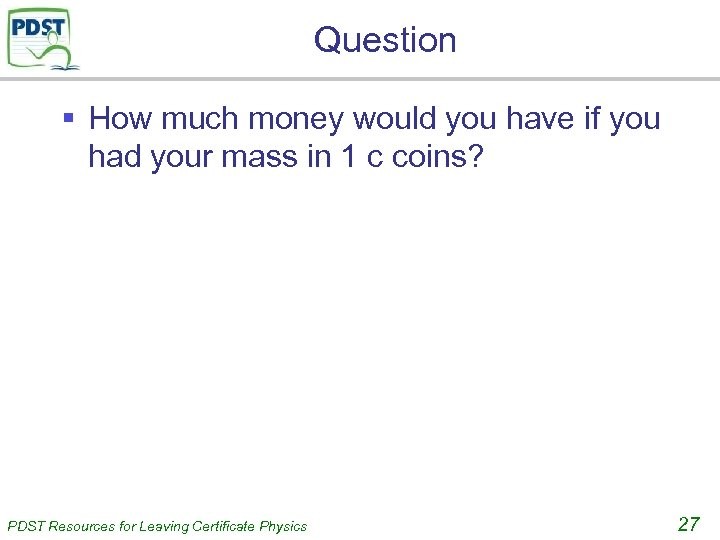Question § How much money would you have if you had your mass in 1 c coins? PDST Resources for Leaving Certificate Physics 27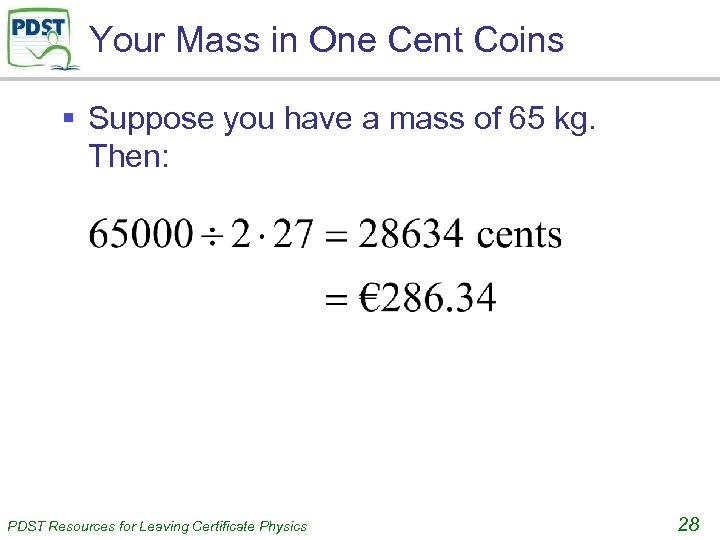Your Mass in One Cent Coins § Suppose you have a mass of 65 kg. Then: PDST Resources for Leaving Certificate Physics 28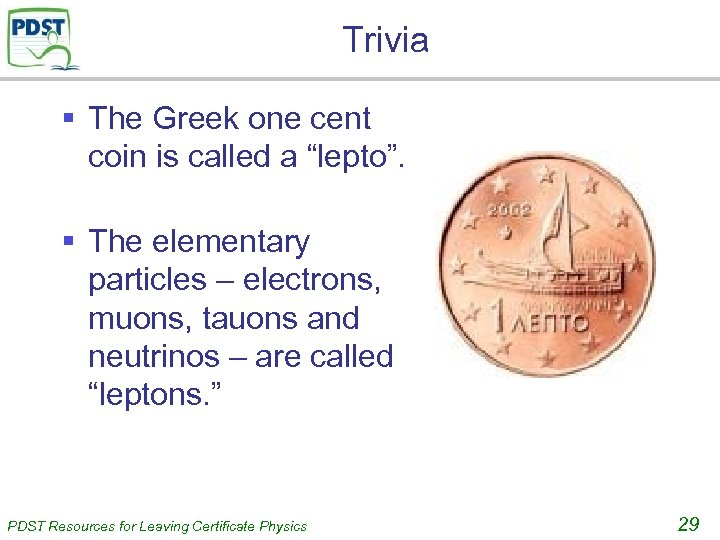Trivia § The Greek one cent coin is called a “lepto”. § The elementary particles – electrons, muons, tauons and neutrinos – are called “leptons. ” PDST Resources for Leaving Certificate Physics 29